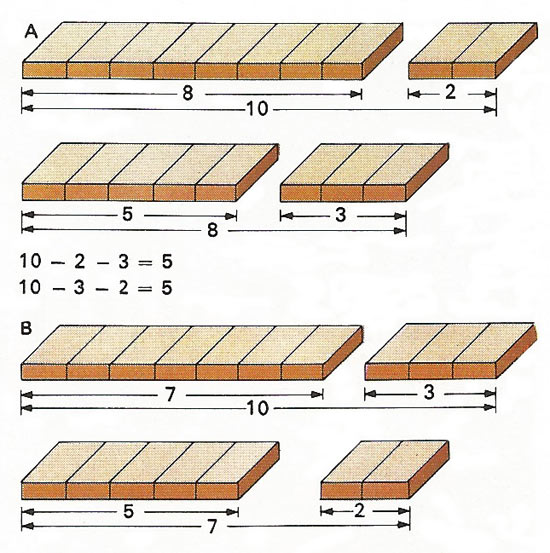A# subtractionSubtraction like addition, is associative – that is, a series of subtractions can be carried out in any order. Starting with a piece of wood 10 units long [A], we can cut off first 2 units and then remove a further 3 units from the 8 remaining (so completing the sum 10 – 2 – 3 = 5). Or [B] we can remove first 3 units and then cut off another 2 units from the original 10. This time the subtraction sum is 10 – 3 – 2 = 5 but the result is exactly the same.

Subtraction is the binary operation of finding the difference between two quantities or numbers; it is the inverse of addition. The difference of two numbers, ab, is the number that has to be added to b to give a. a is called the minuend and b the subtrahend. Subtraction can also be seen as the solution of an equation since the difference (a – b) may be expressed as b + x = a. The subtraction of negative numbers is equivalent to addition, since a – (–b) = a + b.